Function Repository Resource:

# LassoPoints

Create Graphics primitives of a closed curve that encloses a set of points

Contributed by: Jon McLoone
 ResourceFunction["LassoPoints"][pts] return Graphics primitives for a curve that encloses the given 2D points.

## Details and Options

ResourceFunction["LassoPoints"] generates the convex hull of the given points and then uses these as the key points for constructing the curve out of Line and Circle primitives.
The function accepts the option Padding, with the default around points being Scaled[.2].

## Examples

### Basic Examples (2)

 In:=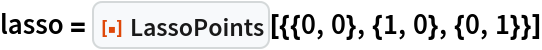Out=In:=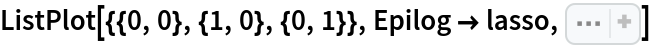Out=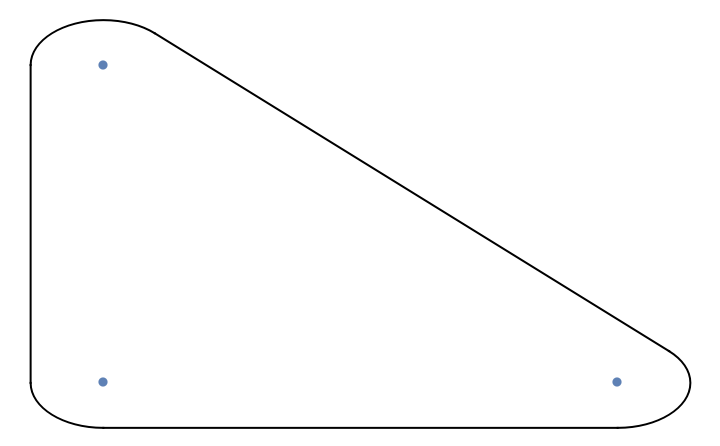Lasso the seven points nearest the mean of some data:

 In:=Out=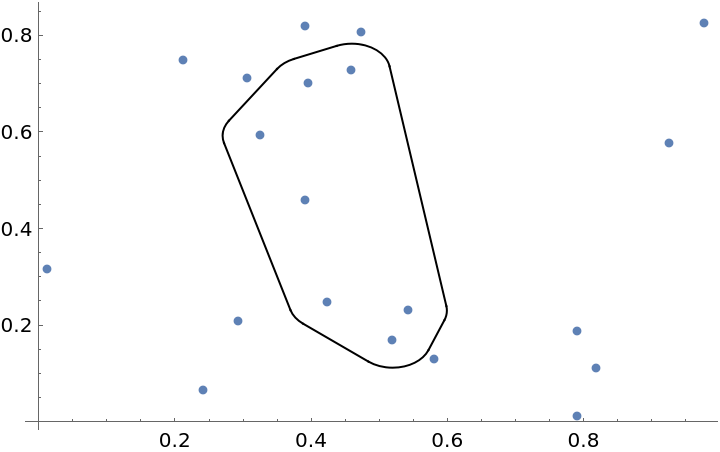### Scope (1)

Lassoing a single point creates a circle:

 In:=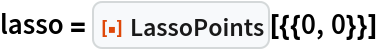Out=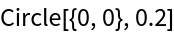In:=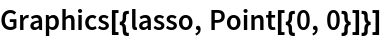Out=### Options (3)

Control how closely the curve encloses the points with Padding:

 In:=Out=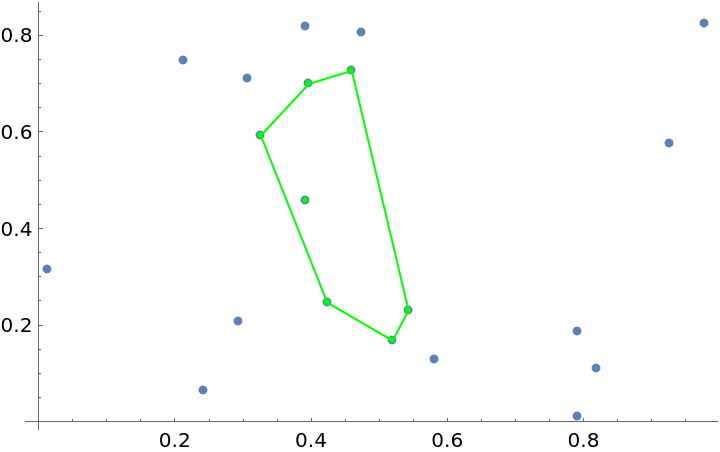Scaled values for Padding represent a proportion of the distance from the centroid of the enclosed area:

 In:=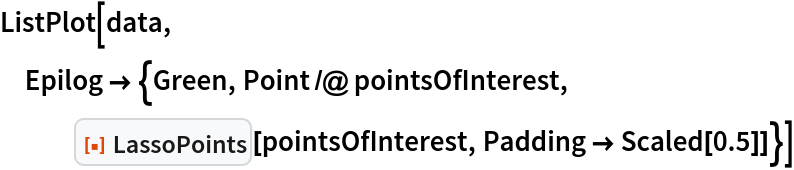Out=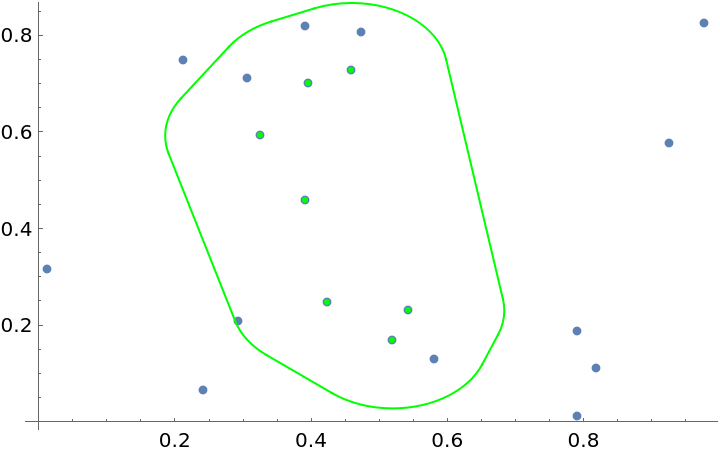Absolute values are applied using the coordinate system of the points:

 In:=Out=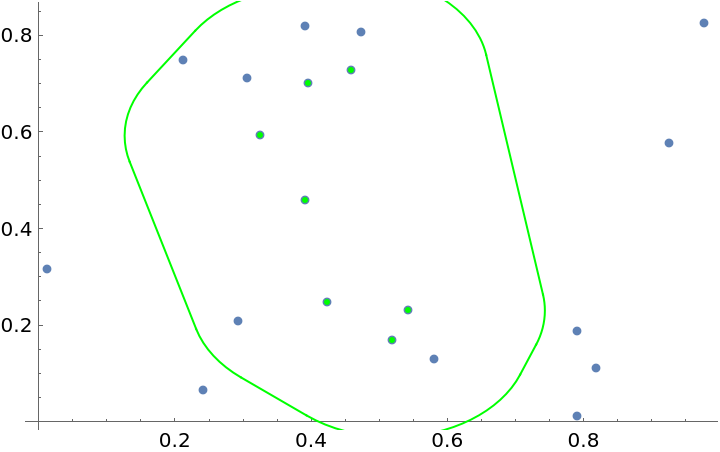Jon McLoone

## Version History

• 1.0.0 – 30 January 2023